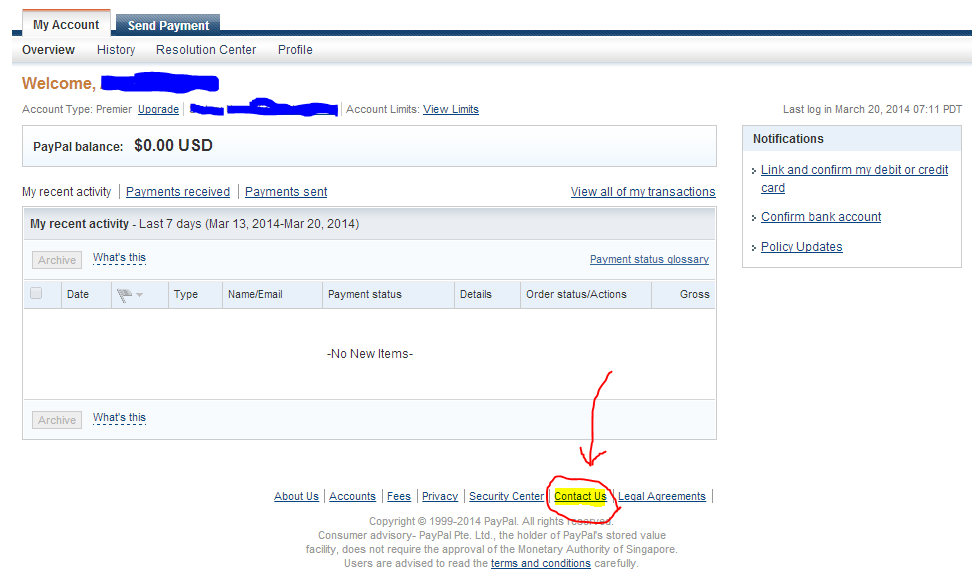# What is Array, One Dimensional Array and Two Dimensional Array in Visual Basic?

## Array, One Dimensional Array and Two Dimensional Array

### Array:

Array is a powerful data structure, which can hold related or unrelated data at consecutive location. In Visual Basic arrays are of one dimension or more than one dimension. But we often use one-dimensional array to present data in vector form and on two-dimensional array when we stores data in matrix form.### Declaration of One Dimensional Array:

One-Dimensional array may be declared as static or dynamic.

#### Static Array Declaration:

In static declaration size of array is fixed at the time of declaration of such array like.

Dim a(4) As Integer

Memory representation  of array "a" will be as under.

0                                1                              2                             3                                4

Above memory representation shows that total number of elements of array "a" are 5 with lower limit 0 and upper limit 4. Like scalar variable if type of array is not defined then default type will be variant, e.g.
Dim b(4)

In Visual Basic index Number of first element may be other than 0. e.g.

Dim c(-2 To 2) As String

Memory representation of array "c" will be as under.

-2                           -1                               0                               1                               2

#### Dynamic Array Declaration:

In dynamic declaration of array, size of array can be changed at any stag. Dynamic array is declared as under.

Dim d() As Integer

Before used of array d, you must define its scope by using Redim statement.e.g.

Redim d(4)

Redim is also used to change the size of array. e.g.

Redim d(7)

When Redim statement is used with dynamic array then data previously stored in array is lost i.e Redim statement resets all values as empty or zero.
Note that an order to avoid the loss of already stored data in array you must use the keyword Preserve with Redim statement like the following examples.

Redim Preserve d(7)

Example:

Start Visual Basic with a new standard EXE project and place two CommandButtons on a Form and set the Caption property of Command1 as "Used Static Array" and Caption property of Command2 as "Used Dynamic Array". and write the following code in the click events of both the CommandButton controls.

 Private Sub Command1_Click()        Dim a(4) As Integer, i As Integer        For I = 0 To 4              A(i) = I = 5        Next i        Print “Out put of static array is”        For i = 0 To 4              Print a(i)        Next iEnd Sub

 Private Sub Command1_Click()        Dim a() As Integer, n As Integer, m As Integer, i As Integer         n = 3         Redim a(n-1)         For i = 0 To n-1                A(i) = i + 5          Next i          M = 2          Redim Preserve a (m+n-1)          For i = n to m + n – 1                 a(i) = i + 5          Next i          Print “Out put of Dynamic Array is”          For i = 0 to m + n -1                 Print a(i)         Next iEnd Sub

Press F5 to run the project and click the "Used of Static array" button to see the output of static array, and click the button "Used of Dynamic Array" to see the output of dynamic array.

### Declaration of Two Dimensional Array:

Two-Dimensional array is declared as aunder:

Dim a(2, 2) As Integer

This declaration of two dimensional array 'a' represent that array has three rows and three columns.

Example:

Start Visual Basic with new Standard EXE project, place a CommandButton on a Form and write following code in the Click event of the CommandButton control.

 Private Sub Command1_Click()        Dim a(2, 2) As Integer, r As Integer, c As Integer        For r = 0 To 2        For c = 0 To 2              a(r, c) = r + c        Next c, r        For r = 0 To 2        For c = 0 To 2              Print a(r, c)        Next c        Next rEnd Sub

Press F5 key to run this project and Click the Button. All the values of two-dimensional array will be displayed on the Form.

## Me : If you need more help, want to ask feel free to use below comment box. It will be my pleasure to help you. THANKS for reading.

This post first appeared on Online Learning, please read the originial post: here

# Share the post

What is Array, One Dimensional Array and Two Dimensional Array in Visual Basic?

×